## Math 480 Course Notes -- June 4, 1996

### Examples of Stochastic processes continuous in time, discrete in space

The simplest useful example of a stochastic process that is continuous in time but discrete in space is the Poisson process. The Poisson process is a special case of a set of processes called birth processes. In these processes, the sample space for each random variable X(t) is the set of non-negative integers, and for each non-negative integer x, we choose a positive real parameter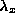which will be equal to both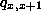and. Thus, in such a process, the value of X can either jump up by 1 or stay the same. The time until the process jumps up by one, starting from X=x, is exponentially distrubuited with parameter. So if we think of X as representing a population, it is one that experiences only births (one at a time) at random times, but no deaths.

The specialization in the Poisson process is thatis chosen to have the same value,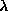, for all x. Since we move one step to the right (on the x-axis) according to the exponential distribution, we can conclude without much thought that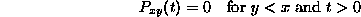and that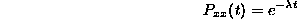for all t>0. To learn any more, we must consider the system of differential equations (the "forward equations") derived in the preceding lecture.

Recall that the forward equationgave the rate of change in the probability of being at y (given that the process started at x) in terms of the probabilities of jumping to y from any z just at time t (the terms of the sum for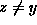) and (minus) the probability of already being at y and jumping away just at time t (recall that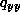is neagtive.

For the Poisson process, the forward equations simplify considerably, because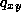is nonzero only if x=y or if y=x+1. And for these values we have: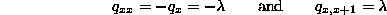Therefore the forward equation reduces to: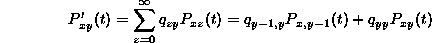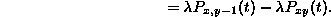We interpret this equation as saying that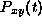changes by increasing due to births from state y-1, and decreases due to births starting from state y. Since we already knowfor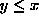, we will use the preceding differential equation inductively to calculatefor y> x, one y at a time.

To do this, though, we need the fact that the solution of the linear first-order differential equation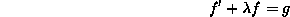isUsing this formula for the solution of the differential equation, we substitutefor f(t), and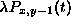for g(t). We get that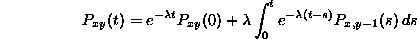Since we are assuming that the process starts at x, i.e., X(0)=x, we know that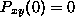for all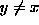and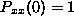. In other words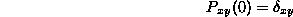(this is the Kronecker delta).

Now we are ready to compute. Note that we have to computefor all possible nonnegative integer values of x and y. In other words, we have to fill in all the entries in the table: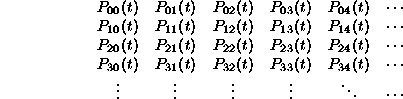Of course, we already know part of the table: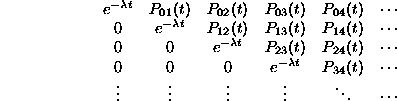So we begin the induction:

We know thatso we can use the differential equation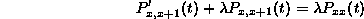to get that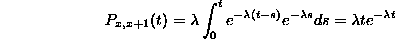Note that this fills in the next diagonal of the table.

Then we can use the differential equation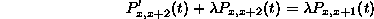to get that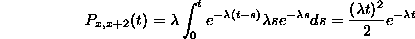Continuing by induction (exercise 1 below!) we get that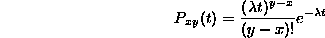provided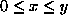and t>0. This means that the random variable X(t) (this is the infinite discrete random variable that gives the value of x at time t, i.e., tells how many jumps have happened up until time t), has a Poisson distribution with parameter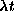This is the important connection between the exponential distribution and the Poisson distribution.

Homework

1. Do the induction proof -- show thatprovidedand t> 0 given the work above.

2. Draw some graphs of the distribution of X(t) for various values of t (and). What happens as t increases?

3. Since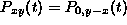for all positive t, show that if X(0)=x, then the random variable X(t)-x has a Poisson distribution with parameter. Then, use the Markov assumption to prove that X(t)-X(s) has Poisson distribution with parameter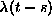.

4. Let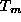be the continuous random variable that gives the time to the mth jump of a Poisson process with parameter. Find the pdf of.

5. For a Poisson process with parameter, find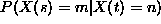, both for the case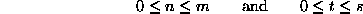and the case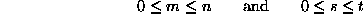Dennis DeTurck
Fri Jun 7 16:34:24 EDT 1996